International
Tables for
Crystallography
Volume H
Powder diffraction
Edited by C. J. Gilmore, J. A. Kaduk and H. Schenk

International Tables for Crystallography (2018). Vol. H, ch. 2.3, pp. 67-70

## Section 2.3.2. Neutrons and neutron diffraction – pertinent details

C. J. Howarda* and E. H. Kisia

aSchool of Engineering, University of Newcastle, Callaghan, NSW 2308, Australia
Correspondence e-mail:  chris.howard@newcastle.edu.au

### 2.3.2. Neutrons and neutron diffraction – pertinent details

| top | pdf |

#### 2.3.2.1. Properties of the neutron

| top | pdf |

The basic properties of the neutron are summarized in Table 2.3.1.

 Table 2.3.1| top | pdf | Properties of the neutron (adapted from Kisi & Howard, 2008)
 Mass (m) 1.675 × 10−27 kg Charge 0 Spin ½ Magnetic moment (μn) −1.913 μN Wavelength (λ) h/mv Wavevector (k) Magnitude 2π/λ Momentum (p)Energy (E)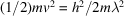#### 2.3.2.2. Neutron scattering lengths

| top | pdf |

The scattering lengths of most interest in neutron powder diffraction are those for coherent elastic scattering, bcoh, often abbreviated to b. As already mentioned, there is no angle (Q) dependence, since the scattering from the nucleus is isotropic. A selection of scattering lengths for different isotopes and different elements is given in Table 2.3.2.

 Table 2.3.2| top | pdf | Coherent scattering lengths and absorption cross sections (for 25 meV neutrons) for selected isotopes
 Data are taken from Section 4.4.4of Volume C (Sears, 2006). Where not stated, the values are for the natural isotopic mix. The X-ray atomic form factors, f, evaluated at Q = 1.2π Å−1, are included for comparison.
ElementIsotopebcoh (fm)σs(tot) (10−24cm2)σa (10−24cm2)fIsotopic abundance (%)
H   −3.7390 (11) 82.02 (6) 0.3326 (7) 0.25
1 −3.7406 (11) 82.03 (6) 0.3326 (7)   99.985
2 6.671 (4) 7.64 (3) 0.000519 (7)   0.015
3 4.792 (27) 3.03 (5) 0

B   5.30 (4) − 0.213 (2)i 5.24 (11) 767 (8) 1.99
10 −0.1 (3) − 1.066 (3)i 3.1 (4) 3835 (9)   20.0
11 6.65 (4) 5.78 (9) 0.0055 (33)   80.0

C   6.6460 (12) 5.551 (3) 0.00350 (7) 2.50
12 6.6511 (16) 5.559 (3) 0.00353 (7)   98.90
13 6.19 (9) 4.84 (14) 0.00137 (4)   1.10

O   5.803 (4) 4.232 (6) 0.00019 (2) 4.09

Ti   −3.370 (13) 4.06 (3) 6.43 (6) 13.2
46 4.725 (5) 2.80 (6) 0.59 (18)   8.2
47 3.53 (7) 3.1 (2) 1.7 (2)   7.4
48 −5.86 (2) 4.32 (3) 8.30 (9)   73.8
49 0.98 (5) 3.4 (3) 2.2 (3)   5.4
50 5.88 (10) 4.34 (15) 0.179 (3)   5.2

V   −0.3824 (12) 5.10 (6) 5.08 (2) 14.0

Ni   10.3 (1) 18.5 (3) 4.49 (16) 18.7
58 14.4 (1) 26.1 (4) 4.6 (3)   68.27
60 2.8 (1) 0.99 (7) 2.9 (2)   26.10
61 7.60 (6) 9.2 (3) 2.5 (8)   1.13
62 −8.7 (2) 9.5 (4) 14.5 (3)   3.59
64 −0.37 (7) 0.017 (7) 1.52 (3)   0.91

Cu   7.718 (4) 8.03 (3) 3.78 (2) 19.9
63 6.43 (15) 5.2 (2) 4.50 (2)   69.17
65 10.61 (19) 14.5 (5) 2.17 (3)   30.83

Zn   5.680 (5) 4.131 (10) 1.11 (2) 20.8

Zr   7.16 (3) 6.46 (14) 0.185 (3) 27.0

Gd   6.5 (5) 180 (2) 49700 (125) 45.9
155 6.0 (1) − 17.0 (1)i 66 (6) 61100 (400)   14.8
157 −1.14 (2) − 71.9 (2)i 1044 (8) 259000 (700)   15.7

Pb   9.405 (3) 11.118 (7) 0.171 (2) 60.9

The first thing to note is the variation in scattering length from element to element and indeed from isotope to isotope. The scattering lengths are in most cases positive real numbers, in which case there is a phase reversal of the neutron on scattering, but for some isotopes the scattering lengths are negative, so there is no change in phase on scattering. The scattering lengths are determined by the details of the neutron–nucleus interaction (Squires, 1978).5 In the event that the neutron–nucleus system is close to a resonance, such as it is for 10B, 155Gd and 157Gd, scattering lengths will be complex quantities and the scattered neutron will have some different phase relationship with the incident one. The imaginary components imply absorption, which is reflected in the very high absorption cross sections, σa, for these isotopes.

The total scattering cross section, σs, is given by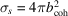when only coherent scattering from a single isotope is involved, which is very nearly the case for oxygen since 99.76% of naturally occurring oxygen is zero-spin 16O. In most cases there is a more substantial contribution from incoherent scattering, which may be either spin or isotope incoherent scattering. Spin incoherent scattering arises because the scattering length depends on the relative orientation of the neutron and nuclear spins, parallel and antiparallel arrangements giving rise to scattering lengths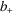and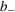, respectively. Isotope incoherent scattering arises because of the different scattering of neutrons from different isotopes of the same element. In almost all circumstances (except, for example, at the extraordinarily low temperatures mentioned in Section 2.3.1) the distributions of spins and isotopes are truly random, which means that there is no angle dependence in this scattering: this is sometimes described as Laue monotonic scattering.

When b varies from nucleus to nucleus (even considering just a single element), the coherent scattering is determined by the average value of b, that is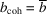,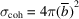, and the average incoherent cross section is given by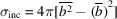. The total scattering cross section σs is the sum of the two cross sections (Squires, 1978; see also Section 2.3.2 in Kisi & Howard, 2008). For the particular case of a nucleus with spin I, the states I + 1/2 and I − 1/2 give scattering determined byand, respectively, and have multiplicities 2I + 2 and 2I, respectively, from which it follows that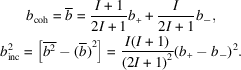More information, including a comprehensive listing of scattering lengths, can be found in Section 4.4.4of International Tables for Crystallography Volume C (Sears, 2006). This listing presents the spin-dependent scattering lengths via bcoh and binc as just defined. Other compilations can be found in the Neutron Data Booklet (Rauch & Waschkowski, 2003), and online through the Atominstitut der Österreichischen Universitäten, Vienna, at http://www.ati.ac.at/~neutropt/scattering/table.html . In addition, the majority of computer programs used for the analysis of data from neutron diffraction incorporate, for convenience, a list of bcoh values for the elements.

#### 2.3.2.3. Refractive index for neutrons

| top | pdf |

The coherent scattering lengths of the nuclei determine the refractive index for neutrons through the relationship (Squires, 1978)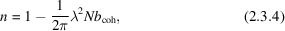where N is the number of nuclei per unit volume. For elements with positive values of the coherent scattering length the refractive index is slightly less than one, and that leads to the possibility of total external reflection of the neutrons by the element in question. In fact, when the coherent scattering length is positive, neutrons will undergo total external reflection for glancing angles less than a critical angle γc given by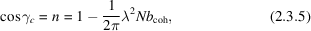which, since γc is small, reduces to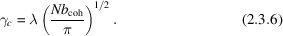It can be seen that the pertinent material quantity is Nbcoh, the coherent scattering length density'; for materials comprising more than one element this is the quantity that would be computed. Since the critical angle for total external reflection is proportional to the neutron wavelength, it is convenient to express this as degrees per ångstrom of neutron wavelength. These are important considerations in the design and development of neutron guides (Section 2.3.3.4).

#### 2.3.2.4. Neutron attenuation

| top | pdf |

Neutron beams are attenuated by coherent scattering, incoherent scattering and true absorption. The cross sections for all these processes are included in the tables cited above. For powder diffraction, the coherent scattering is usually small because it takes place only in that small fraction of crystallites correctly oriented for Bragg reflection; the other processes, however, take place throughout the sample.

If a particular scattering entity i with scattering cross sections (σi)inc and (σi)abs is present at a number density Ni, then the contribution it makes to the linear attenuation coefficient μ is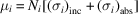. If the mass is Mi, then the density is simply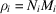, so we have the means to evaluate the mass absorption coefficient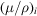. The calculation of absorption for elements, compounds and mixtures commonly proceeds by the manipulation of mass absorption coefficients, in the same manner as is employed for X-rays (see Section 2.4.2 in Kisi & Howard, 2008).

#### 2.3.2.5. Magnetic form factors and magnetic scattering lengths

| top | pdf |

For a complete treatment of the magnetic interaction between the neutron and an atom carrying a magnetic moment, and the resulting scattering, the reader is referred elsewhere [Marshall & Lovesey, 1971; Squires, 1978; Section 6.1.2of Volume C (Brown, 2006a)]. The magnetic moment of an atom is associated with unpaired electrons, but may comprise both spin and orbital contributions. The magnetic interaction between the neutron and the atom depends on the directions of the scattering vector and the magnetic moment vector according to a triple vector product. The direction of polarization of the neutron must also be taken into account. For an unpolarized incident beam, the usual case in neutron powder diffraction, it is a useful consequence of the triple vector product that the magnetic scattering depends on the sine of the angle that the scattering vector makes with the magnetic moment on the scattering atom (see Section 2.3.4 and Chapter 7 in Kisi & Howard, 2008). The extent of the unpaired electron distribution (usually outer electrons) implies that the scattering diminishes as a function of Q, an effect that can be described by a magnetic form factor. For a well defined direction for the magnetic moment M, and with a distribution of moment that can be described by a normalized scalar m(r), the form factor as a function of the scattering vector h [defined in equation (1.1.17)in Chapter 1.1]6 is the Fourier transform of m(r),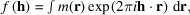where m(r) can comprise both spin and orbital contributions [Section 6.1.2of Volume C (Brown, 2006a)]. The tabulated form factors are based on the assumption that the electron distributions are spherically symmetric, so that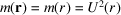, where U(r) is the radial part of the wave function for the unpaired electron. In the expansion of the plane-wave functionin terms of spherical Bessel functions, we find that the leading term is just the zeroth-order spherical Bessel function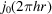with a Fourier transform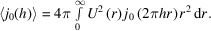This quantity is inherently normalized to unity at h = 0, and may suffice to describe the form factor for spherical spin-only cases. In other cases it may be necessary to include additional terms in the expansion, and these have Fourier transforms of the form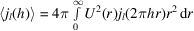with l even; these terms are zero at h = 0 (Brown, 2006a). In practice these quantities are evaluated using theoretical calculations of the radial distribution functions for the unpaired electrons [Section 4.4.5of Volume C (Brown, 2006b)].

Form factors can be obtained from data tabulated in Section 4.4.5of Volume C (Brown, 2006b). Data are available for elements and ions in the 3d- and 4d-block transition series, for rare-earth ions and for actinide ions. These data are provided by way of the coefficients of analytical approximations to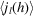, the analytical approximations being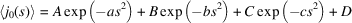and for l ≠ 0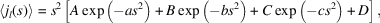where s = h/2 in Å−1. These approximations, with the appropriate coefficients, are expected to be coded in to any computer program purporting to analyse magnetic structures. Although the tabulated form factors are based on theoretical wave functions, it is worth noting that the incoherent scattering from an ideally disordered (i.e., paramagnetic) magnetic system will display the magnetic form factor directly.

It is often convenient to define a (Q-dependent) magnetic scattering length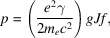where me and e are the mass and charge of the electron, γ (= μn) is the magnetic moment of the neutron, c is the speed of light, J is the total angular momentum quantum number, and g is the Landé splitting factor given in terms of the spin S, orbital angular momentum L, and total angular momentum quantum numbers by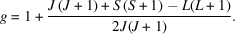For the spin-only case, L = 0, J = S, so g = 2. The differential magnetic scattering cross section per atom is then given by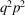where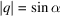, α being the angle between the scattering vector and the direction of the magnetic moment. This geometrical factor is very important, since it can help in the determination of the orientation of the moment of interest; there is no signal, for example, when the moment is parallel to the scattering vector. Further discussion appears in Chapters 2 (Section 2.3.4) and 7 in Kisi & Howard (2008).

#### 2.3.2.6. Structure factors

| top | pdf |

The locations of the Bragg peaks for neutrons are calculated as they are for X-rays7 (Section 1.1.2), and the intensities of these peaks are determined by a structure factor, which in the nuclear case is [cf. Chapter 1.1, equation (1.1.56)]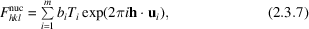where bi here denotes the coherent scattering length, Ti has been introduced to represent the effect of atomic displacements (thermal or otherwise, see Section 2.4.1 in Kisi & Howard, 2008), h is the scattering vector for the hkl reflection, and the vectors ui represent the positions of the m atoms in the unit cell.

For coherent magnetic scattering, the structure factor reads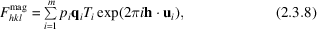where pi is the magnetic scattering length. The vector qi is the magnetic interaction vector' and is defined by a triple vector product (Section 2.3.4 in Kisi & Howard, 2008), and has modulus sin α as already mentioned. In this case the sum needs to be taken over the magnetic atoms only.

As expected by analogy with the X-ray case, the intensity of purely nuclear scattering is proportional to the square of the modulus of the structure factor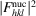. In the simplest case of a collinear magnetic structure and an unpolarized incident neutron beam, the intensity contributed by the magnetic scattering is proportional to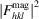, and the nuclear and magnetic contributions are additive.

### References

Brown, P. J. (2006a). Magnetic scattering of neutrons. International Tables for Crystallography, Volume C, Mathematical, Physical and Chemical Tables, 1st online ed., edited by E. Prince, pp. 590–593. Chester: International Union of Crystallography.Google Scholar
Brown, P. J. (2006b). Magnetic form factors. International Tables for Crystallography, Volume C, Mathematical, Physical and Chemical Tables, 1st online ed., edited by E. Prince, pp. 454–461. Chester: International Union of Crystallography.Google Scholar
Kisi, E. H. & Howard, C. J. (2008). Applications of Neutron Powder Diffraction. Oxford University Press.Google Scholar
Marshall, W. & Lovesey, S. W. (1971). Theory of Thermal Neutron Scattering: The Use of Neutrons for the Investigation of Condensed Matter. Oxford: Clarendon Press.Google Scholar
Rauch, H. & Waschkowski, W. (2003). Neutron scattering lengths. Neutron Data Booklet, 2nd ed., edited by A. J. Dianoux & G. Lander, §1.1. Grenoble: Institut Laue–Langevin.Google Scholar
Sears, V. F. (2006). Scattering lengths for neutrons. International Tables for Crystallography, Volume C, Mathematical, Physical and Chemical Tables, 1st online ed., edited by E. Prince, pp. 444–454. Chester: International Union of Crystallography.Google Scholar
Squires, G. L. (1978). Introduction to the Theory of Thermal Neutron Scattering. Cambridge University Press.Google Scholar